# Electrostatics Notes for IIT JEE

Electrostatics is a branch of physics that deals with static electricity. The study of electrostatics requires the concept of electric charge. Electric charge is the inherent property of certain fundamental particles because of which particles experience a force when it is placed in an electromagnetic field. It is a scalar quantity as the charge has only magnitude and no direction. The charges can be either positive or negative. It is represented by the symbol e

e = 1.6 x 10-19 coulomb

## Properties of Charge

1) Unlike charges attract each other while like charges repel each other.

2) Charge is a scalar quantity.

3) Charge will not exist without mass, while mass can exist without charge. Particles with zero rest mass like the photons or neutrino will not have any charge.

4) While charging, the mass of the body will change. If the electrons are removed the mass of the body will decrease and if the electrons are added the mass of the body will increase. Due to the extremely small mass of electrons, the change in the mass of the body is negligible.

5) Charge is transferable. When an uncharged body is placed near a charged body, the charge gets transferred from the uncharged body to the charged body.

6) Electric charges only appear in discrete amounts, it is said to be quantized.

7) Charge is conserved. For an isolated charge, the total charge will remain constant, the charge is neither created nor destroyed, it is transferred from one body to another.

8) Charge is invariant. This means that the charge will not change on the body with speed.

9) A charged particle at rest will produce an electric field. The accelerated charges will radiate energy in the form of electromagnetic waves and produce an electric and magnetic field.

10) The SI unit for charge is Coulomb.

11) A body can be charged by friction, induction or radiation.

## Coulomb’s Law

Statement

Coulomb’s law states that the force of interaction of two stationary point charges is directly proportional to the product of the magnitude of the charges and inversely proportional to the square of the distance between them.

Explanation

Consider two point charges q1 and q2 at rest. Let d be the separation between them. According to Coulomb’s law, the force between the two charges is

F = kq1q2/d2

Where k is the positive constant of proportionality. The value of k depends on the medium in which the charges are situated and the system of units.

If the two charges are placed in a vacuum, then the value of k is given as

k = (1/4πε0) = 8.9875 x 109 = 9 x 109 Nm2C-2

ε0 is the permittivity of the free space.

Therefore, the Coulomb force acting between two point charges placed in the vacuum is

$F_{0}=\frac{1}{4\pi \epsilon _{0}}\frac{q_{1}q_{2}}{d^{2}}$ ——(1)

If the two charges are placed in the material medium, then

k = (1/4πε)

ε is the absolute permittivity of the medium.

Therefore, the Coulomb force acting between two point charges placed in the medium with the absolute permittivity ε is

$F=\frac{1}{4\pi \epsilon}\frac{q_{1}q_{2}}{d^{2}}$———-(2)

From equation (1) and (2) we get

$\frac{F_{0}}{F}=\frac{\epsilon }{\epsilon _{0}}=\epsilon _{r}$

εr is the relative permittivity or the dielectric constant of the medium

Coulomb’s Law in Vector Form

The direction of the force acting between two charges depends on their nature and it is along the line joining the centre of two charges.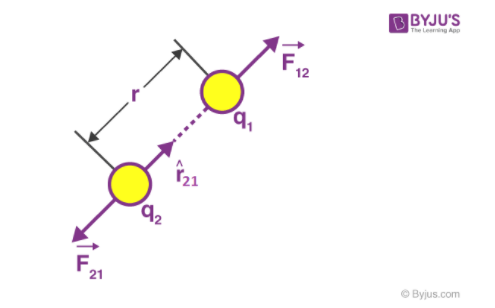The force on q2 due to q1 is

$\vec{F_{21}}=\frac{1}{4\pi \epsilon _{0}\epsilon _{r}}\frac{q_{1}q_{2}}{r_{12}^{2}}\hat{r_{12}}$

The force on q1 due to q2 is

$\vec{F_{12}}=\frac{1}{4\pi \epsilon _{0}\epsilon _{r}}\frac{q_{1}q_{2}}{r_{21}^{2}}\hat{r_{21}}$

$\vec{F_{12}}= – \vec{F_{21}} (as\, \hat{r_{12}}=-\hat{r_{21}})$

Or $\vec{F_{12}} + \vec{F_{21}} = 0$

## Comparison of Electrostatic Force and Gravitational Force

Two charged bodies will experience both electrostatic force and the gravitational force on account of their masses.

Similarities

1. Both are central forces. They act along the line joining the two forces.
2. They observe inverse square law i.e., they vary inversely with the square of the distance between the two interacting bodies.
3. They are conservative forces. The work done is independent of the path chosen.
4. Both the forces can operate in the vacuum as well as material media between the interacting bodies.

Dissimilarities

1. Gravitational force is between two masses while electrostatic force is between the two charged particles.
2. Electrostatic force can either be attractive or repulsive while gravitational force is always attractive.
3. The electrostatic force is not universal while gravitational force is a universal force.
4. The electrostatic force is much stronger than the gravitational force.

## Electric Field

The space surrounding a charge or a system of charges in which the other charged particles experience electrical forces is called the electrostatic field or electric field.

Electrostatic Field Strength

The strength of the electric field is measured by the force experienced by a unit positive charge placed at that point. The direction of the field is given by the direction of the unit positive charge if it were free to move.

$\vec{E}=\frac{\vec{F}}{q}$

### Electric Field Due to Various Charge Distribution

 Isolated point charge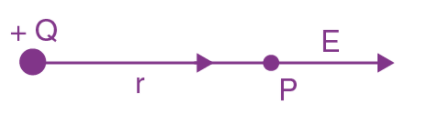$E = \frac{1}{4\pi \epsilon _{0}}\frac{q}{r^{2}}\hat{r}$ Dipole (i)At a point on the axial line $E = \frac{1}{4\pi \epsilon _{0}}\frac{2p}{d^{3}}$ (ii) At a point on the equatorial line $E = \frac{1}{4\pi \epsilon _{0}}\frac{p}{d^{3}}$ (iii) At a general point $E = \frac{1}{4\pi \epsilon _{0}}\frac{p}{d^{3}}\sqrt{3cos^{2}\theta +1}$ Here, p is the dipole moment Ring of uniform charge distribution $E = \frac{1}{4\pi \epsilon _{0}}\frac{qx}{(a^{2}+x^{2})^{3/2}}$ Spherical Shell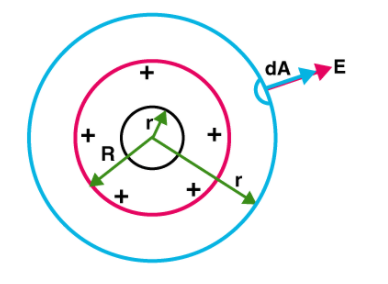Outside shell, $E_{out}= \frac{1}{4\pi \epsilon _{0}}\frac{q}{r^{2}}$ Inside Shell, Ein = 0 Cylindrical conductor of infinite length having line charge λ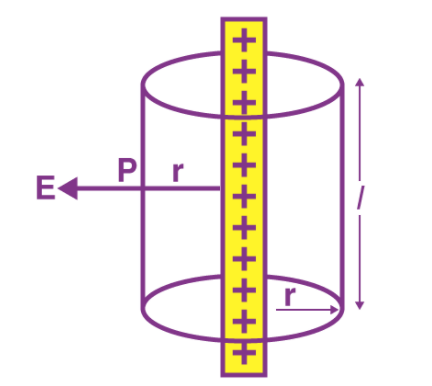Outside cylinder, E = λ/2πε0r Inside cylinder, E = 0

## Electric Dipole

A system consisting of two equal and opposite point charges separated by a small distance is called an electric dipole.

Dipole Moment

The dipole moment is the product of one of the charges and the vector distance from the negative to positive charge.

$\vec{P}=q\vec{a}$

### Dipole in an External Uniform Field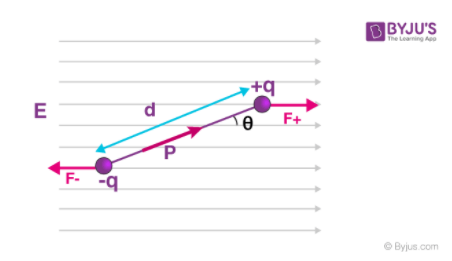If the dipole is oriented at an angle θ to a uniform electric field as shown in the figure, the charges experience equal and opposite forces. So there is no net force on the dipole. However, there is a net torque on the dipole.

$\vec{\tau }=\vec{p}\times \vec{E}$

The magnitude of the torque is τ =pEsin θ

## Electric Field Lines

An electric field line is an imaginary path along which a unit positive charge which is free to move travels.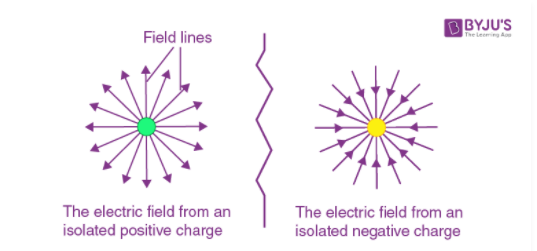1. The direction of the electric field is always tangent to the electric field lines or the lines of force.
2. The lines of force are directed towards an isolated negative charge and are directed away from the isolated positive charge.
3. The lines of force will start at the positive charge and end at the negative charge.
4. The number of field lines leaving a positive charge and terminating at a negative charge is proportional to the magnitude of the charge.
5. The electric field lines will never intersect.
6. Electric field lines will never form closed loops
7. In a uniform electric field, the electric field lines are equidistant and parallel to each other.

## Gauss Theorem

The Gauss theorem states that for any charge distribution, the total electric flux linked with a closed surface is 1/ε0 times the total charge enclosed by the surface.

Mathematically, $\oint \vec{E}.\vec{ds}=\frac{q}{\epsilon _{0}}$

Electric Flux

The electric flux through a surface is defined as the number of lines of force passing normally through the surface. The flux per unit area of the surface at any point in an electric field is the measure of the electric intensity at that point.

## Electric Potential

The electric potential at a point in an electric field is defined as the amount of work done in moving a unit positive charge from infinity to the point against the electric field. The SI unit of potential is Volt.

Relation between electric field and electric potential

The electric field E = – (dV/dx)

dV/dx is the rate of change of potential with distance.

### Electric Potential Due to Various Charge Distribution

 Point Charge V = (1/4πε0)(q/r) Several Charges V = (1/4πε0)(q1/r1+q2/r2+q3/r3) Charged Spherical Shell Vout = (1/4πε0)(q/r) Vin = – (1/4πε0)(q/R) On the surface, VSurface = (1/4πε0)(q/R) Electric dipole (i) point on the axial line, V = p/4πε0r2 (ii) point on the equatorial line , V = 0 Uniformly charged non conducting sphere (i) Outside, Vout = (1/4πε0)(q/r) (ii) Inside, Vin = (1/4πε0)(q(3R2-r2/2R3) (iii) On the surface, Vsurface = (1/4πε0)(q/R) (iv) In centre, Vcentre = (3/2)(1/4πε0)(q/R)

### Electrostatic Potential Energy

The electrostatic potential energy of a system of a point charge is defined as the total amount of work done in bringing the individual charges from their state of rest at infinity to constitute the system.

Case (1) Electric Potential Energy For Two Point Charges.

W = U = (1/4πε0)(q1q2/r12) = q1V1

Case (2) Electric Potential Energy for Three-Point Charges

W = U = (1/4πε0)(q1q2/r12 + q1q3/r13 + q2q3/r23)

## Capacitor

A capacitor is an electrical device for storing a large quantity of charge and hence electrical energy. A capacitor in the simplest form consists of two parallel conductors of any arbitrary shape separated by a dielectric medium in between

Capacitance of the Capacitor

The capacitance of the capacitor is defined as the ratio of the magnitude of the charge on either conductor to the potential difference between the conductors forming the capacitor.

C = Q/V

The capacitance of the capacitor depends on the

1. Size and shape of the conductors
2. The separation between the conductors
3. The dielectric medium used between the conductors

The SI unit of capacitance is Farad

Dielectrics

Dielectrics are insulators. They do not have any free electrons and hence will not conduct any electricity. However, they greatly affect the electric field in which they are placed. The molecules of the dielectric are of two types: polar molecules and nonpolar molecules. Some of the examples of dielectrics are vacuum, mica, air, paper etc.

Dielectric strength of a Dielectric Medium

When a dielectric is placed in a very high electric field, the outer electrons get detached from the parent atoms. They become free electrons. Under this condition, dielectrics behave like a conductor. Charges are conducted through the conductor. This phenomenon is called dielectric breakdown. The maximum value of the applied dielectric field above which dielectric breakdown occurs is called the ‘dielectric strength’. The dielectric strength is measured in kilovolt per millimetre.

Definition of Dielectric Constant and Relative Permittivity

The dielectric constant or relative permittivity of a medium can be defined as the ratio of the electrostatic force between the two-point charges placed in the vacuum to the electrostatic force between the same two point charges separated by the same distance in the medium.

### Principle of Capacitor

Let an insulated metal plate A be given a positive charge till its potential becomes maximum. When another insulated plate B is kept near A then the inner face of B becomes negatively charged and the outer face of B will be positively charged. The negative charge of B will try to reduce the potential of A and the positive charge of B will try to increase it. When the outer side of B is earthed, the positive charges will flow to earth while the negative charge will stay and further reduce the potential of A. Thus, a larger amount of charge can be given to A to raise it to maximum potential.

### Capacitance of a Spherical Capacitor

A spherical capacitor consists of two hollow concentric spherical conductors The inner conductor of radius Rin is charged positively and the outer conductor of radius Rout is charged negatively.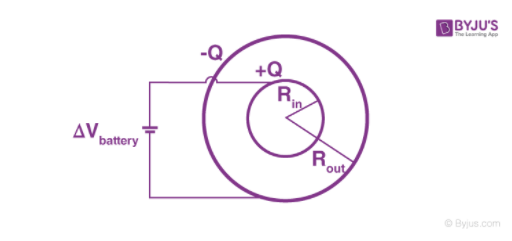If air is the dielectric medium then the capacitance of the capacitor is

$C = 4\pi \epsilon _{0}\frac{R_{1}R_{2}}{R_{2}-R_{1}}$

If the medium between the two conductors is of relative permittivity εr, then

$C = 4\pi \epsilon _{0}\epsilon _{r}\frac{R_{1}R_{2}}{R_{2}-R_{1}}$

From the above expression, it can be understood that the capacitance of the capacitor may be increased by

1. Using spherical conductors of larger radii
2. Decreasing the separation between the conductors
3. Using a medium of a higher dielectric constant between the two conductors

### Capacitance of a Parallel Plate Capacitor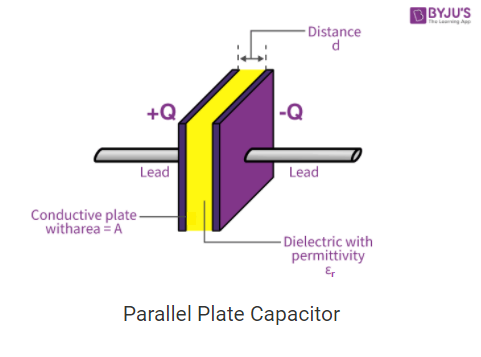The parallel plate capacitor is the simplest and commonly used type of capacitor. It consists of two large identical plane parallel conductors of area A separated by a distance d.

The capacitance of a parallel plate air capacitor is

C = ε0A/d

If the dielectric medium is of relative permeability εr then capacitance becomes

C = εrε0A/d

### Capacitance of a Cylindrical Capacitor

A cylindrical capacitor consists of two coaxial cylindrical conductors. The inner cylindrical conductor of radius ‘a’ is charged positively to an extent + Q. It induces an equal amount of negative charge – Q on the outer cylindrical conductor of radius ‘b’ which is earthed. If ‘l’ is the length of the cylindrical capacitor and the medium between the conductors is air, then the capacitance of the capacitor is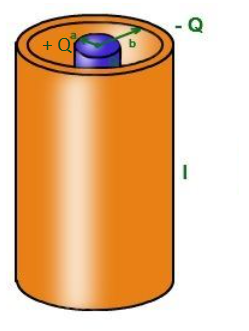$C=\frac{2\pi \epsilon _{0}l}{2.303log(b/a)}$

If the medium between the two conductors is of relative permittivity εr then

$C=\frac{2\pi\epsilon _{r} \epsilon _{0}l}{2.303log(b/a)}$

Energy Stored in a Charged Capacitor

The amount of work done in charging the capacitor is stored as electrostatic potential energy U in the capacitor. Therefore, the energy stored in a capacitor charged to an extent Q is

U = Q2/2C = (½) CV2 = (½)QV Joule

Force Between Plates Of Capacitor

The two parallel plates of the capacitor will attract each other because they are oppositely charged.

Electric field due to positive plate E = σ/2ε0

= Q/2ε0 A (Since charge density, σ = Q/A)

Force on the negative charge -Q is F = -QE = Q2/2ε0A

Magnitude of force F = Q2/2ε0 A

= (½) ε0 AE2

## Combination of Capacitors

A set of capacitors can be combined in a number of ways. The two basic combinations of capacitors is series and parallel.

### Capacitors in Series

Two or more capacitors are said to be connected in series when they are connected end to end between two given points so that on applying a potential difference across the combination, the magnitude of the charge on each capacitor is the same. The applied potential difference will be equal to the sum of the resulting potential difference across each capacitor.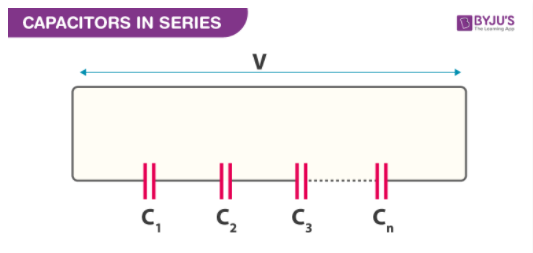The reciprocal of equivalent capacitance of a number of capacitors in series is equal to the sum of the reciprocals of individual capacitances. For n capacitors in series

$\frac{1}{C_{s}}=\frac{1}{C_{1}}+\frac{1}{C_{2}}+\frac{1}{C_{3}}+—-+\frac{1}{C_{n}}$

### Capacitors in Parallel

Two or more capacitors are said to be connected in parallel when they are connected between the same two points so that on applying a potential difference across the combination, the potential difference across each capacitor will be the same.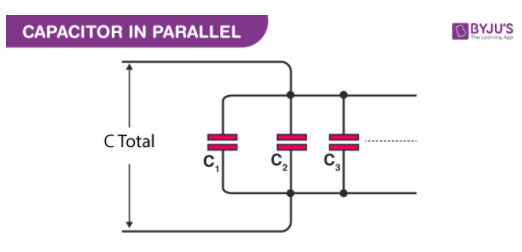The equivalent capacitance of a number of capacitors in parallel is equal to the sum of their individual capacitances.

Cp = C1 + C2 + ——–+ Cn

## Uses of Capacitors

Capacitors have many applications in electrical and electronic circuits. A few applications are listed below

1. To store electric charge and energy
2. It acts as potential dividers
3. In an induction coil, capacitors are used to eliminate sparking
4. In radio circuits for tuning
6. To generate and detect electromagnetic waves.

### Solved Examples

(1) Two identical charges repel each other with a force equal to 10 mg–wt, when they are 0.6 m apart in the air (take, g =10 ms-2 ). The value of each charge is

1)2mC

2)2 x 10-7 C

3) 2 nC

4) 2 μC

Solution:

$F = \frac{1}{4\pi \epsilon _{0}}\frac{q_{1}q_{2}}{r^{2}}$

$\Rightarrow 10 \times 10^{-3}\times 10= \frac{9\times 10^{9}\times q^{2}}{(0.6)^{2}}$

$\Rightarrow q = 2 \times 10^{-6}C$

= 2μC

(2) A charge Q is divided into two parts q and Q – q. What is the value of q for the maximum force between them?

1) 3Q/4

2) Q/3

3) Q

4) Q/2

Solution:

From Coulomb’s law,

F = Kq(Q -q)/R2

F = (K/R2)(Qq – q2)

Adding and subtracting (Q/2)2 and rearranging

F = (K/R2)(- {q2– Qq+(Q/2)2)+(Q/2)2)

F = (K/R2)(Q2/4 – (q – Q/2)2)

F≤ (KQ2/4R2) and the maximum value occurs when the second term is zero.

Therefore, q = Q/2 for maximum force

(3) Four metal conductors having different shapes

1) a sphere

2) cylinder

3) pear

4) lightning conductor

are mounted on insulating stands and charged. Then which one is best suited to retain the charges for a longer time?

Solution:

In a spherical metal conductor, the charges are uniformly distributed over the entire surface Therefore, it retains the charges for a longer period of time. In the case of non-spherical shapes, the concentration of charge will be different in different points due to which the charges will not stay long on the surface.

(4) If a conducting medium is placed between two charges, then the electric force between them will become

1) Zero

2) infinity

3) 1 N

4) 1 dyne

Solution:

If the conducting medium is placed between two charges, the two charges will behave in such a way that they behave as a single charge. The electrical force acts only when there are two charges. Since the charges act as a single charge the force between them will be zero.

(5) Capacity of a capacitor is 48 μF. When it is charged from 0.1 C to 0.5 C, a change in the energy stored is

1) 2500 J

2) 2.5 x 10-3 J

3) 2.5 x 106 J

4) 2.42 x 10-2 J

Solution:

C = 48 μF

= 48 x 10-6 F

q1 = 0.1 C

q2 = 0.5 C

Energy Stored, U = (½) CV2

Since, Q = CV

U = (½) C(Q/C)2

U = (½) (Q2/C)

Change in energy $\triangle U = \frac{1}{2}\left [ \frac{q_{1}^{2}-q_{2}^{2}}{C} \right ]$

$\triangle U = \frac{1}{2}\left [ \frac{(0.5)^{2}-(0.1)^{2}}{48\times 10^{-6}} \right ]$

$\triangle U = \frac{1}{2}\left [ \frac{(0.25-0.01}{48\times 10^{-6}} \right ]$

ΔU = 2500 J

## Practice Problems

(1) A capacitor of capacitance value 1 µF is charged to 30 V and the battery is then disconnected. If the remaining circuit is connected across a 2µF capacitor. the energy lost by the system is

1) 300 μJ

2) 450 μJ

3) 225 μJ

4) 150 μJ

5) 100 μJ

(2) A battery is used to charge a parallel plate capacitor till the potential difference between the plates becomes equal to the electromotive force of the battery. The ratio of the energy stored in the capacitor and the work done by the battery will be

1) 1: 1

2) 2: 1

3) 1: 4

4) 1: 2

(3) A parallel plate air capacitor is connected to a battery. The quantities charge, voltage, electric field and energy associated with this capacitor are given by Q0, V0, E0 and U0 respectively. A dielectric slab is now introduced to fill the space between the plates with the battery still in connection. The corresponding quantities are now given by Q, V, E and U are related to the previous one as

1) Q＞Q0

2) V ＞V0

3) E ＞E0

4) U ＞U0

(4) Potential and field strength at a certain distance from a point charge is 600 V and 200 NC -1. The distance of the point from the charge is

1) 2 m

2) 4 m

3) 8 m

4) 3 m

(5) The electric charges are distributed in a small volume. The flux of the electric field through a spherical surface of radius 10 cm surrounding the total charge is 20 Vm. The flux over a concentric sphere of radius 20 cm will be

1) 20 Vm

2) 25 Vm

3) 40 Vm

4) 200 Vm

### Why does the electric field in a dielectric decrease when it is placed in an external electric field?

The electric field in the dielectric decreases due to polarisation. Polarisation creates an internal electric field in the dielectric which is opposite in direction to the external electric field. Thus, the net electric field reduces.

### What will happen to the capacitance of the capacitor when a dielectric is placed between the conducting plates of the capacitor.

When a dielectric is introduced between the conducting plates of the capacitor, the electric field strength is reduced inside the capacitor. This results in a smaller voltage difference between the plates of the capacitor. The capacitor will store the same charge for a smaller voltage. This implies that the capacitor will have a larger capacitance because of the dielectric.

### Three capacitors each of capacitance 9pF are connected in series. 1. What is the total capacitance of the combination? 2. What will be the potential difference across each capacitor if the combination is connected to a 120 V supply?

Given:
The three capacitors are of capacitance 9pF
The equivalent capacitance of the capacitors connected is series is given by
$\frac{1}{C_{s}}=\frac{1}{C_{1}}+\frac{1}{C_{2}}+\frac{1}{C_{3}}$ $\frac{1}{C_{s}}=\frac{1}{9}+\frac{1}{9}+\frac{1}{9}$

= 3/9 = ⅓
Therefore, Cs = 3μF

### What is the dielectric breakdown?

The phenomenon by which a dielectric will lose its insulating properties and starts behaving like a conductor is called the dielectric breakdown.

### State Gauss law in electrostatics.

The total flux through any hypothetical surface is equal to (1/ε0) times the net charge enclosed by the surface.